## Airy Projection

A Map Projection. The inverse equations for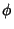are computed by iteration. Let the Angle of the projection plane be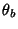. Define(1)

For proper convergence, letand compute the initial point by checking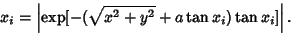(2)

As long as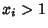, takeand iterate again. The first value for which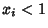is then the starting point. Then compute(3)

until the change in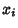between evaluations is smaller than the acceptable tolerance. The (inverse) equations are then given by(4)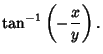(5)